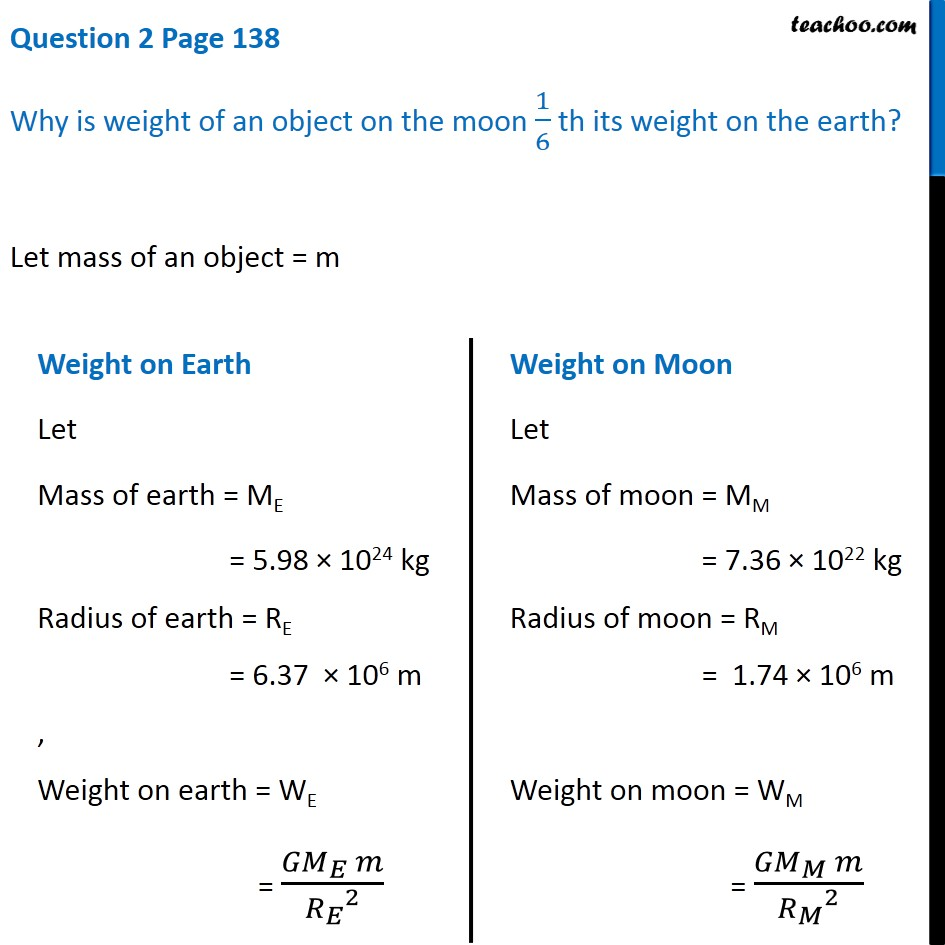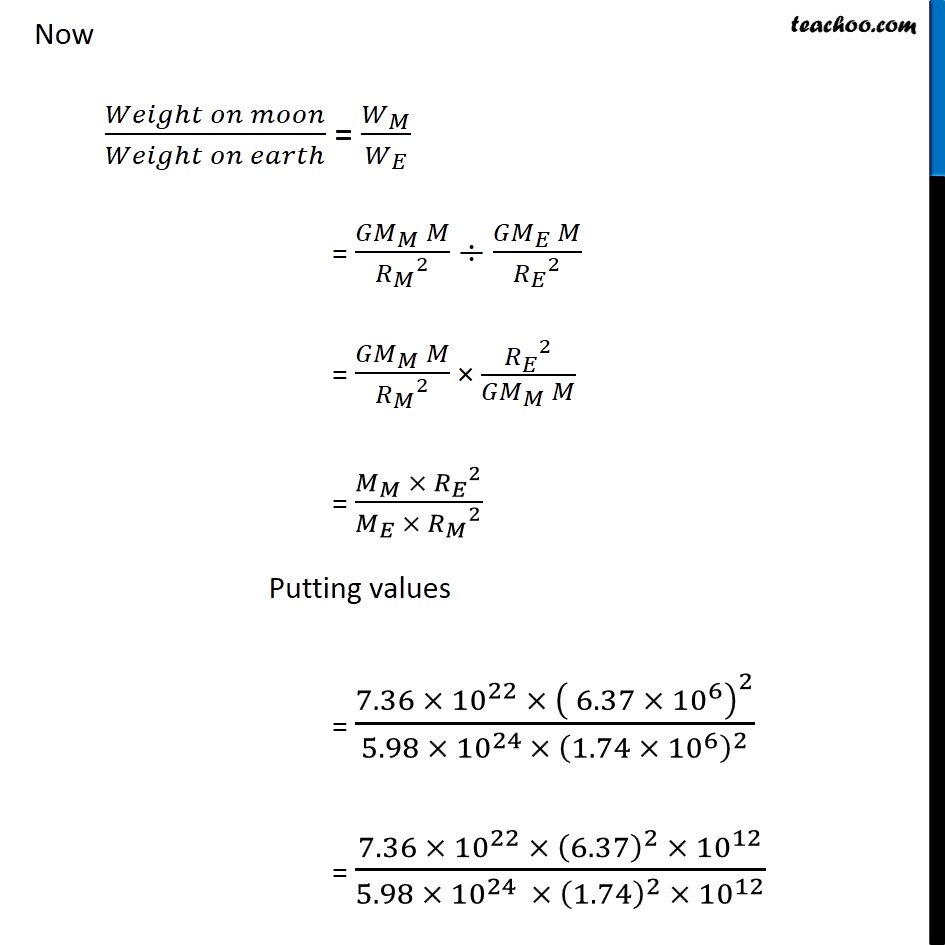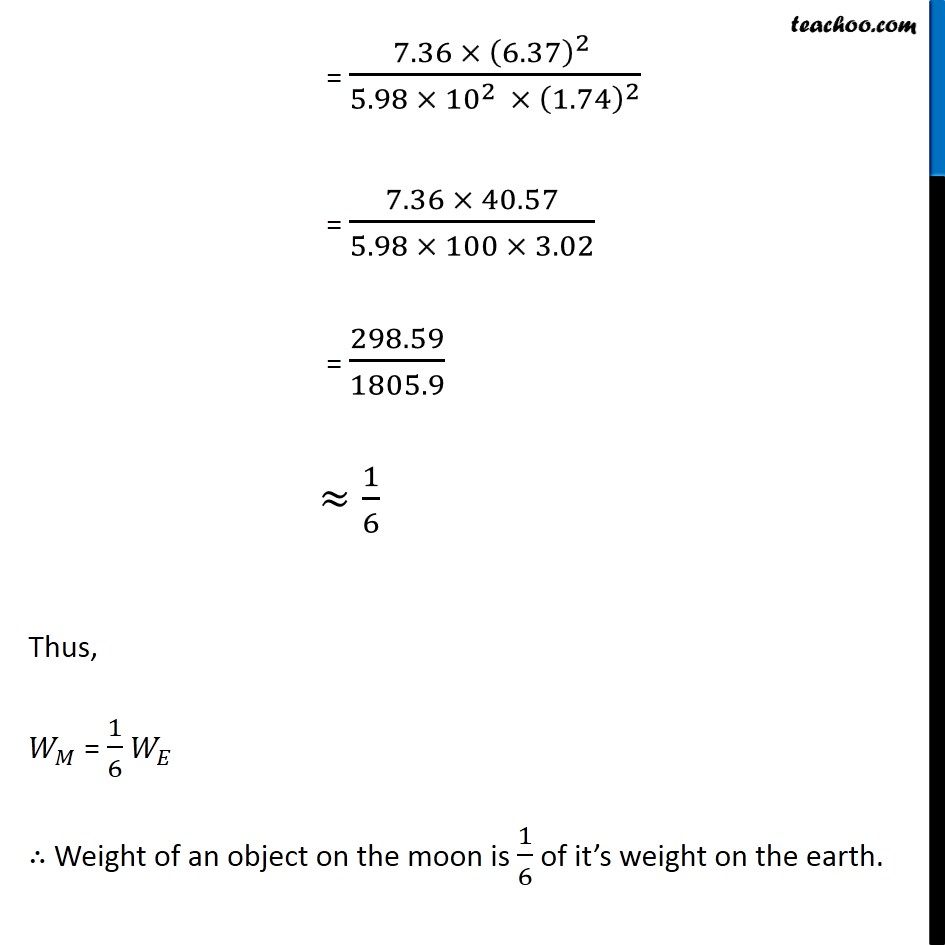Questions from Inside the chapter

Class 9
Chapter 10 Class 9 - GravitationLearn in your speed, with individual attention - Teachoo Maths 1-on-1 Class

### Transcript

Question 2 Page 138 Why is weight of an object on the moon 1/6 th its weight on the earth? Let mass of an object = m Weight on Earth Let Mass of earth = ME = 5.98 × 1024 kg Radius of earth = RE = 6.37 × 106 m , Weight on earth = WE = (〖𝐺𝑀〗_𝐸 𝑚)/〖𝑅_𝐸〗^2 Weight on Moon Let Mass of moon = MM = 7.36 × 1022 kg Radius of moon = RM = 1.74 × 106 m Weight on moon = WM = (〖𝐺𝑀〗_𝑀 𝑚)/〖𝑅_𝑀〗^2 Now (𝑊𝑒𝑖𝑔ℎ𝑡 𝑜𝑛 𝑚𝑜𝑜𝑛)/(𝑊𝑒𝑖𝑔ℎ𝑡 𝑜𝑛 𝑒𝑎𝑟𝑡ℎ) = 𝑊_𝑀/𝑊_𝐸 = (〖𝐺𝑀〗_𝑀 𝑀)/〖𝑅_𝑀〗^2 ÷ (〖𝐺𝑀〗_𝐸 𝑀)/〖𝑅_𝐸〗^2 = (〖𝐺𝑀〗_𝑀 𝑀)/〖𝑅_𝑀〗^2 × 〖𝑅_𝐸〗^2/(〖𝐺𝑀〗_𝑀 𝑀) = (𝑀_𝑀 × 〖𝑅_𝐸〗^2)/(𝑀_𝐸 × 〖𝑅_𝑀〗^2 ) Putting values = (7.36 × 〖10〗^22 × ( 6.37 ×〖 10〗^6 )^2)/(5.98 × 〖10〗^(24 )× (1.74 × 〖10〗^6 )^2 ) = (7.36 × 〖10〗^22 × (6.37)^2 × 〖10〗^12)/(5.98 × 〖10〗^(24 ) × (1.74)^2 × 〖10〗^12 ) = (7.36 × (6.37)^2 )/(5.98 × 〖10〗^(2 ) × (1.74)^2 ) = (7.36 × 40.57)/(5.98 × 100 × 3.02) = 298.59/1805.9 ≈1/6 Thus, 𝑊_𝑀 = 1/6 𝑊_𝐸 ∴ Weight of an object on the moon is 1/6 of it’s weight on the earth.